Learn all Concepts of Chapter 5 Class 10 (with VIDEOS). Check - Arithmetic Progressions - Class 10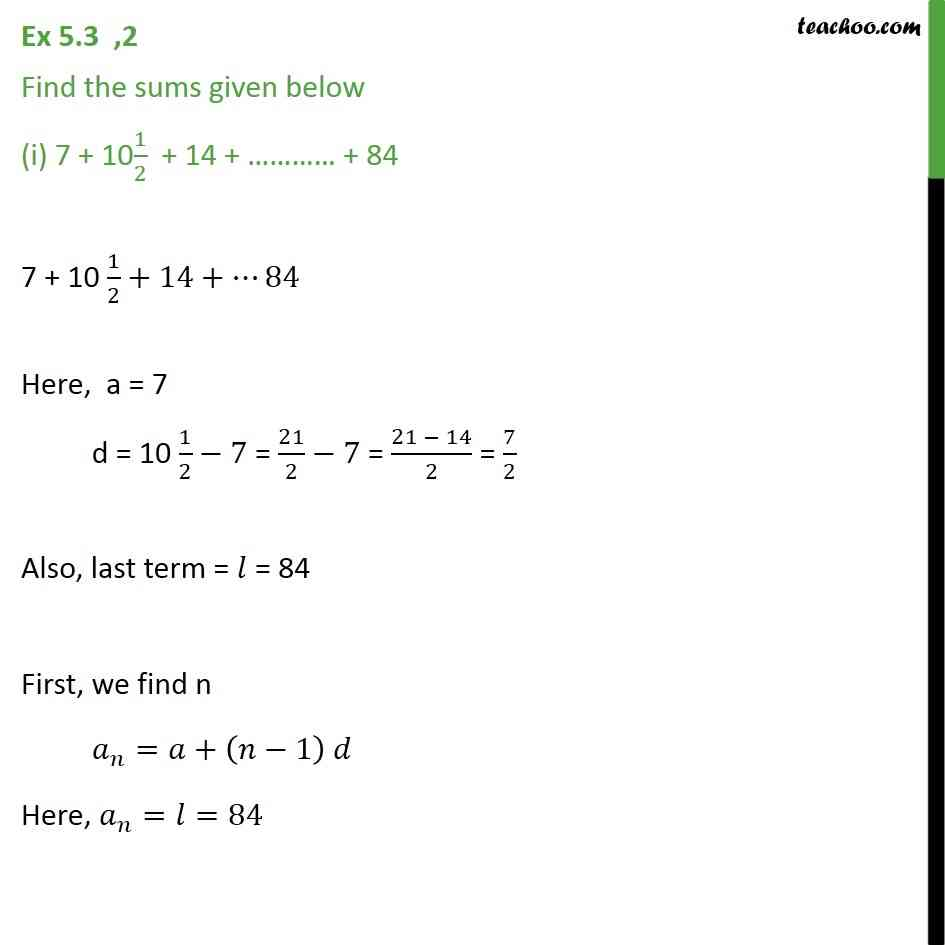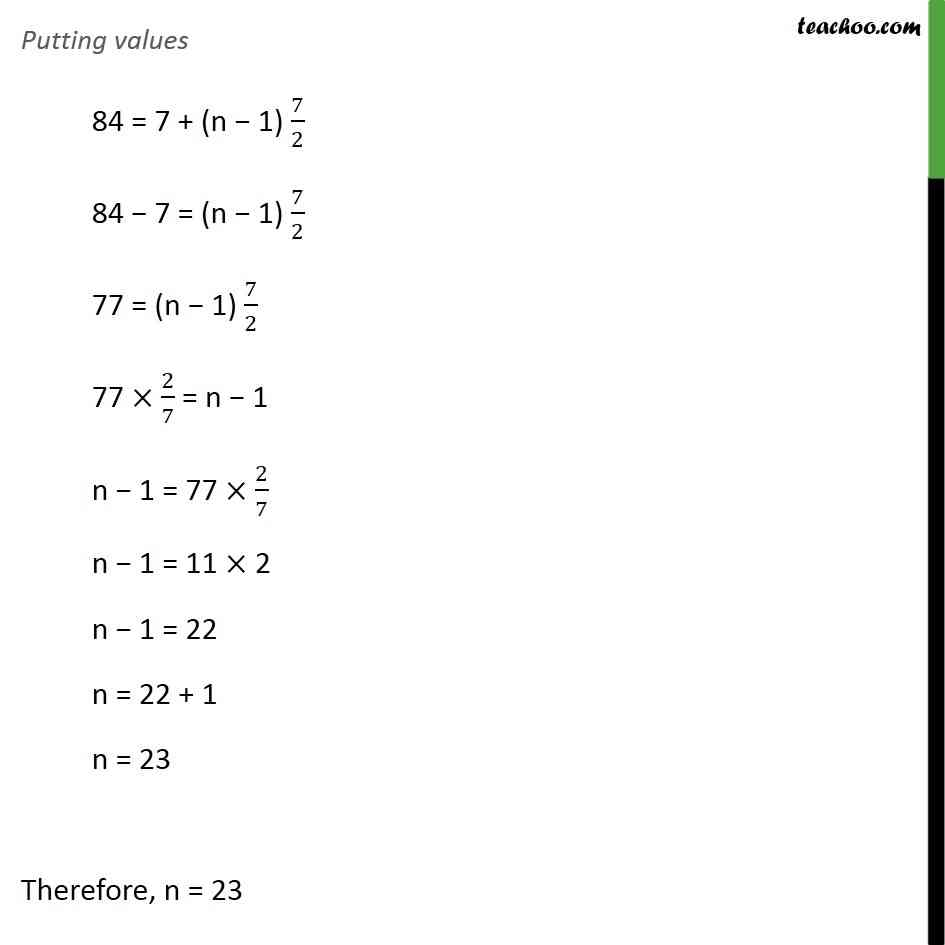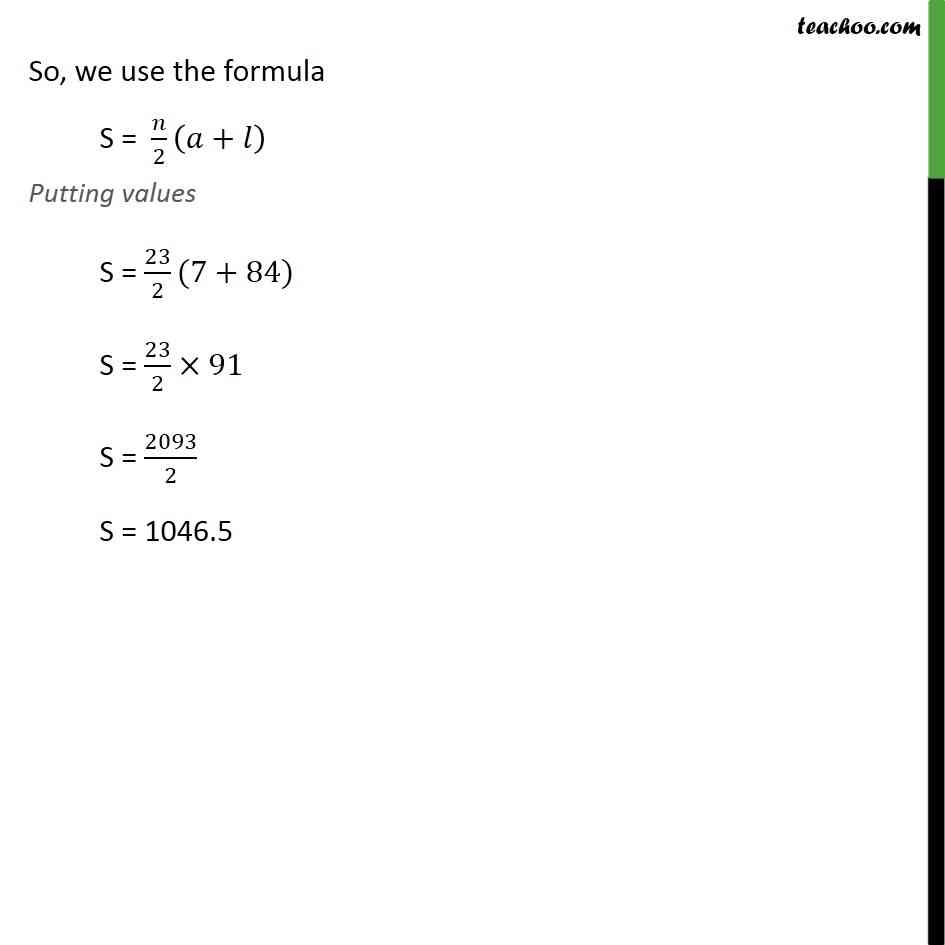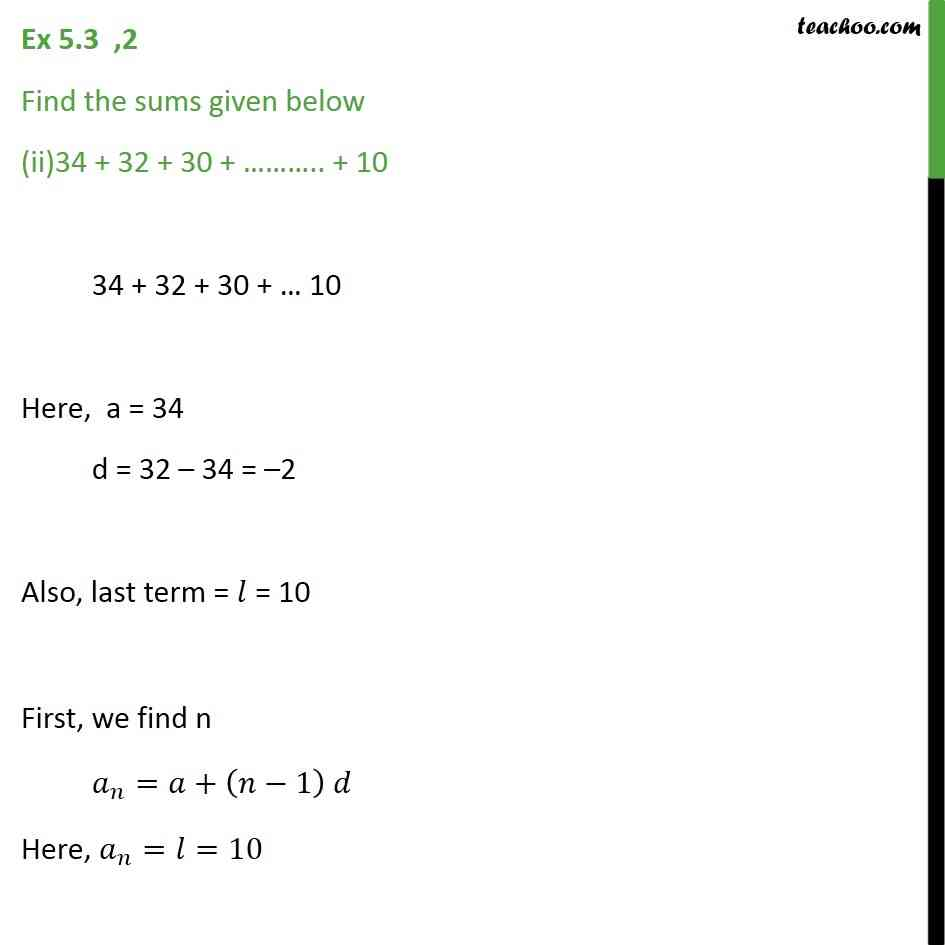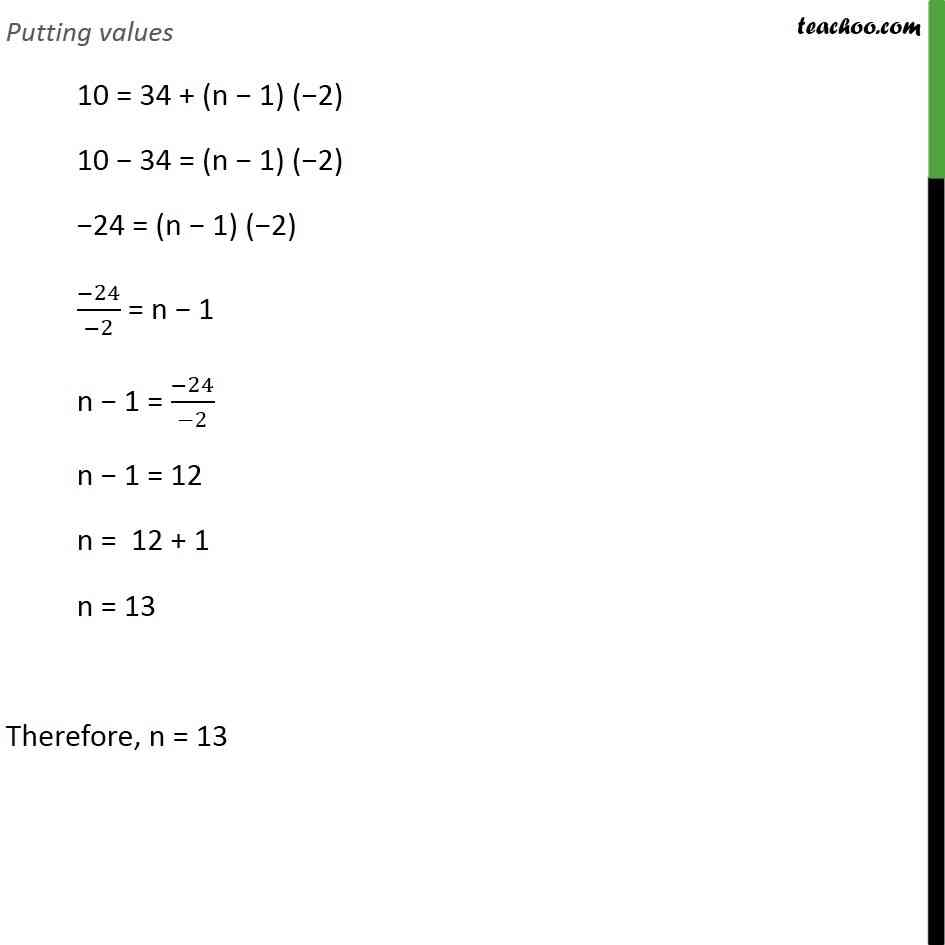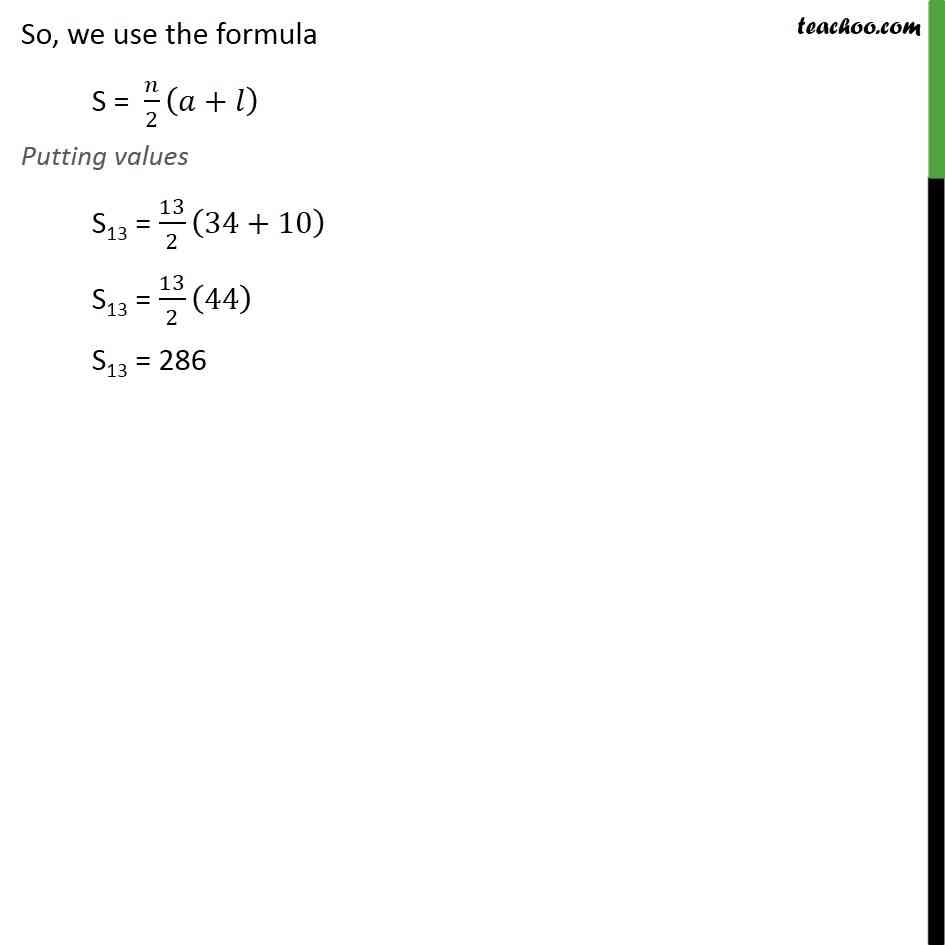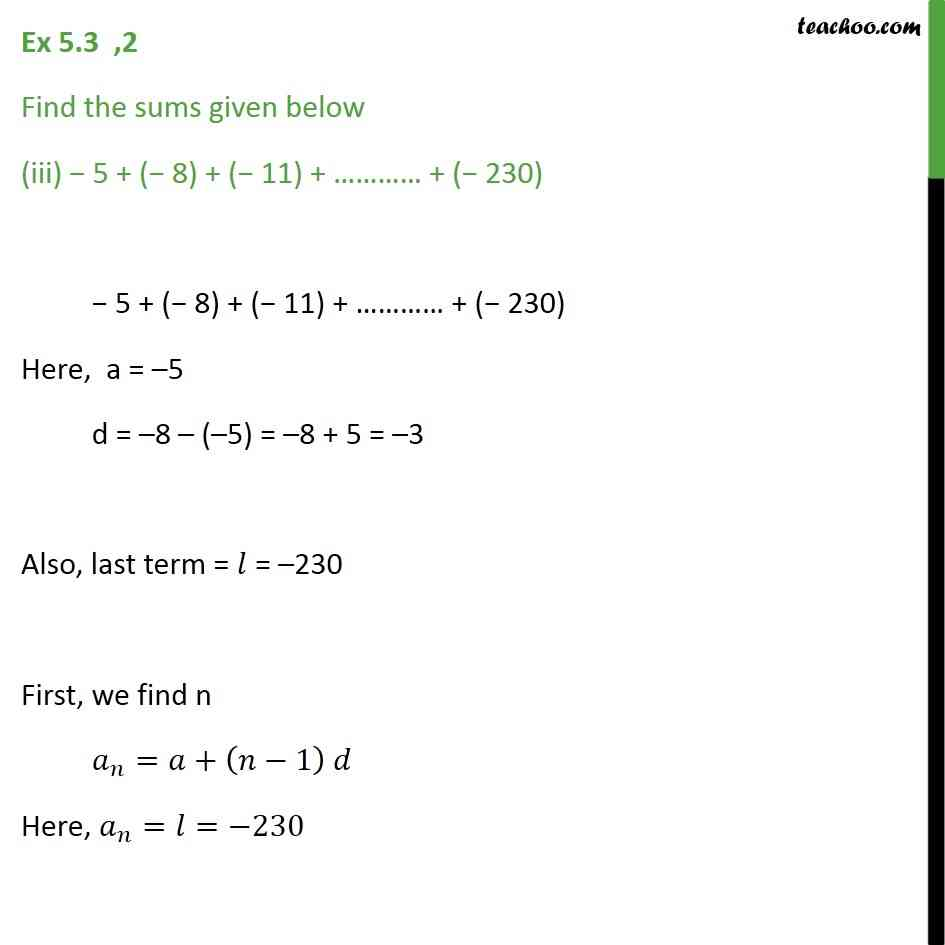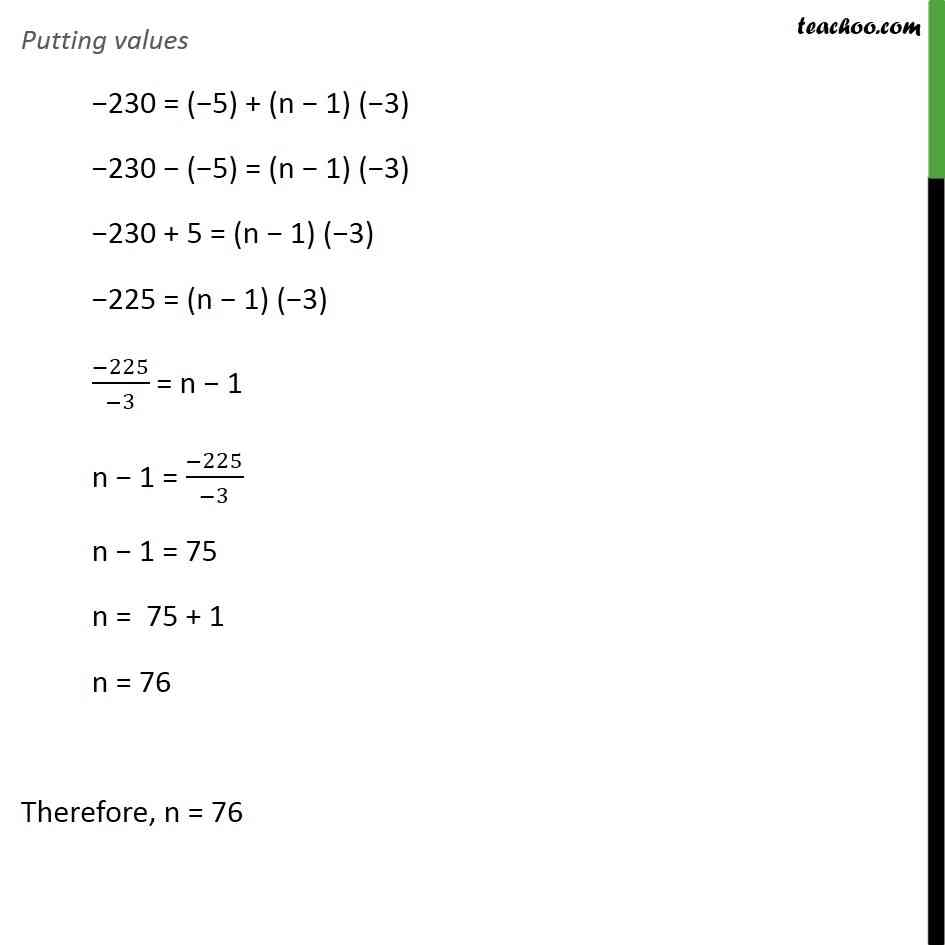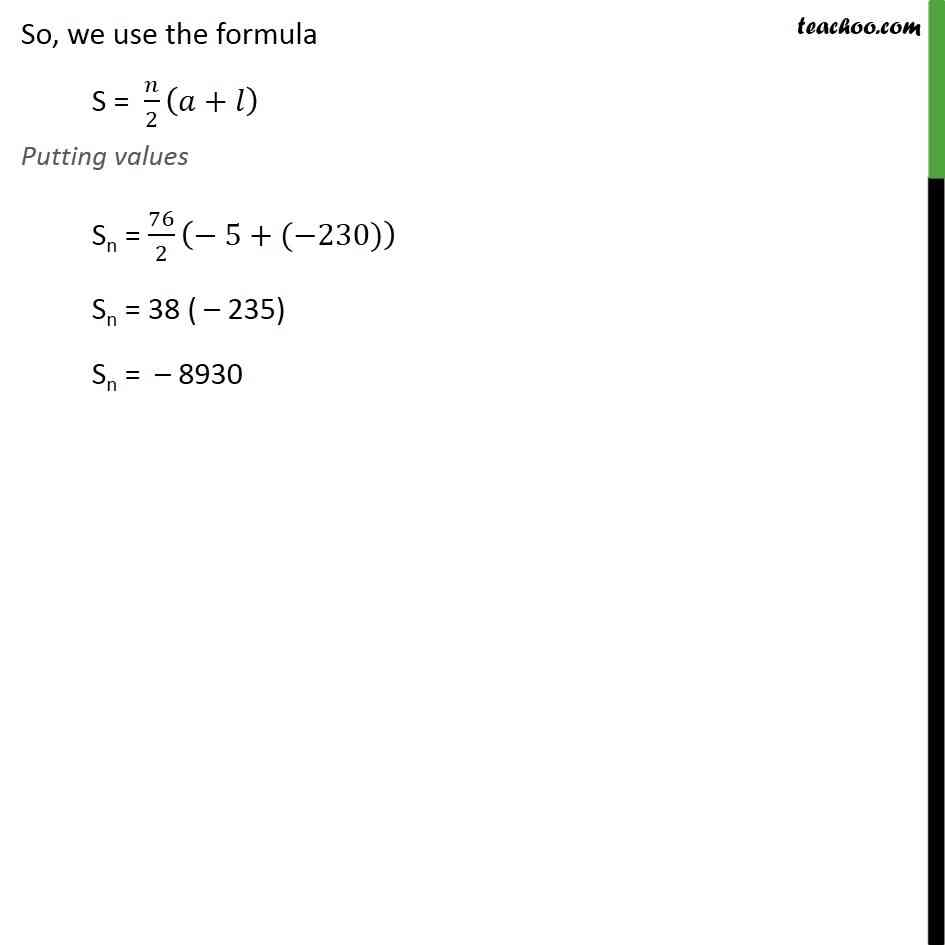1. Chapter 5 Class 10 Arithmetic Progressions
2. Serial order wise
3. Ex 5.3

Transcript

Ex 5.3 ,2 Find the sums given below (i) 7 + 101/2 + 14 + + 84 7 + 10 1/2+14+ 84 Here, a = 7 d = 10 1/2 7 = 21/2 7 = (21 14)/2 = 7/2 Also, last term = = 84 So, we use the formula S = /2 ( + ) Putting values S = 23/2(7+84) S = 23/2 91 S = 2093/2 S = 1046.5 Ex 5.3 ,2 Find the sums given below (ii)34 + 32 + 30 + .. + 10 34 + 32 + 30 + 10 Here, a = 34 d = 32 34 = 2 Also, last term = = 84 So, we use the formula S = /2 ( + ) Putting values S13 = 13/2 (34+10) S13 = 13/2 (44) S13 = 286 Ex 5.3 ,2 Find the sums given below (iii) 5 + ( 8) + ( 11) + + ( 230) 5 + ( 8) + ( 11) + + ( 230) Here, a = 5 d = 8 ( 5) = 8 + 5 = 3 Also, last term = = 230 So, we use the formula S = /2 ( + ) Putting values Sn = 76/2 ( 5+( 230)) Sn = 38 ( 235) Sn = 8930

Ex 5.3